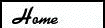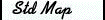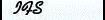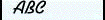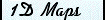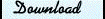#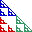Iterated Function SystemsIFS is a Macintosh application for drawing the fractals created by iterated function systems. A standard example is the "Sierpinski gasket" which is the icon for the application. IFS requires MacOS 10.6 or later.

 IFS version 2.2.1 Mac OS X 10.6-10.14 (Intel processor)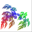Download

What it does

Iterated function systems are dynamical systems defined by a set of n mappings fi. In the case of IFS, we allow the fi to be affine maps of the plane:

f(x) = A x + b

where A =[[a,b],[c,d]] is a 2x2 matrix and b is a two dimensional vector. If 0 <|det(A)| < 1, then f is a contraction, and any point under iteration

x´= f(x)

approaches the unique fixed point x = - A-1 b.

An iterated function system allows the choice of any of the n affine maps with probabilities pi for the iteration, thus

x´ = fi(x) with probability pi

In the program, n is initially 3, and the pi = 1/3, so that it is equally likely to have any one of the fi. An iterated function system with contractions fi has a unique attractor S that satisfies the equation

S = f1(S) U f2(S) U … fn(S)

Often this attractor is fractal in structure. Making pictures of these attractors is the job for IFS.

Using IFS

Choosing a transformation The Sierpinski triangle is defined by the affine transformations fi with A = [[0.5,0,],[0,0.5]], and b1 = (0,0), b2= (0,0.5) and b3 =(0.5,0). To obtain this with the program choose "Sierpinski Relative" from the "Show" menu, and type in "0" for each of the symmetry elements.

The Sierpinski relatives have fi given by the same matrices as before, but composed with one of the 8 symmetry transformations of the square. We let D0 be the identity, D1 be the rotation by 90 degrees, and D4 be the reflection about the x-axis. The group elements are defined as

D0 = I
D1 = [[0,1,],[-1,0]]
D2 = D12,
D3 = D13,
D4 = [[1,0],[0,-1]],
D5 = D4D1,
D6 = D4D2,
D7 = D4D3

With the "Sierpinski relatives" menu, you can choose a symmetry element for each of the fi. This gives 28 possible attractors, of which 232 are distinct structures. There are four distinct topological types among these structures—to learn more about this see [Robins, 1997].

The second way to choose the transformations fi, is to use the "Similarity transformations" menu item. A similarity transformation is a rotation composed with a scaling. To choose each transformation you click twice in the plot window. The first click sets the vector b, which is the iterate of the origin. The second click chooses the position which the point (1,0) iterates to. So your second click chooses the angle through which the x-axis is rotated, as well as the length to which it is scaled. Hold down the option key to reflect the similarity (det(A) < 0). To choose all n-transformations, you must choose two points in the plot window for each.

Finally, you can choose a general affine transformation with the "Affine Transformation" menu item. The affine transformation is fixed by 3 vectors, the two columns of A and the vector b. A first click in the plot window fixes b, the second fixes the first column of A (setting the image of the point (1,0)), and the third click fixes the second column of A (setting the image of the point (0,1)).

The program starts up by displaying the orbit of the origin under 50,000 iterations with randomly chosen fi. We use the basic "srand" function built into C for the pseudo-random number generator, so don’t expect sophisticated statistical properties.

You may also show "recursive" iteration. This means to show the image of the unit square (0,0) x (1,1) under all possible sequences of iterations fi fj fk…, out to some level l. Initially l = 3.

You may also view just the first iterate of the unit square under each of the transformations by selecting the "Show: Affine Transformations" menu item.

Finally, if there is a picture in the clipboard, you may paste it into the plot window, and show this. However, this is not working to well with the other parts of the program yet.

You may set the number of random iterations (N = 50,000 initially), the number of levels of the recursive iteration (L=3 initially), choose the number of transformations, (n=3 initially) and the probabilities pi (pi = 1/n initially) with the "Set Parameters" menu.

Apart from the usual commands,you may zoom in or zoom out of the plot by selecting the appropriate menu items. To zoom, drag a rectangle in the plot window.

You may also choose the colors for each transformation fi in this menu. The colors are used to display the color of each point xt after t iterations according to the simple IFS for colors: color´ = 0.5 (color + color(fi)). Thus if we iterate for a long time with f1, then the color of the point approaches the color of f1.

This is a rudamentary version a MacOS Application, and as such is to be thought of as a rough outline of a complete program. Let me know your thoughts about how the program should improve!

This program is free for noncommercial use, but the copyright remains with the author. It may not be sold or included in any commercial collection without the express written approval of the author.

Known Problems (might be called bugs)

• On some computers, when you drag the resize box, or drag to graphically create an affine transformation, the old rectangles are not completely erased. In some cases the window background goes black. I believe this has something to do with my mixing of OpenGL and Quickdraw graphics calls. But I haven't isolated it yet.

References

Barnsley, M. (1988). Fractals everywhere. Boston, Academic Press.

Peitgen, H. O., H. Jürgens, et al. (1992). Chaos and Fractals. New York, Spinger-Verlag.

Robins, V., J. D. Meiss, et al. (1997). "Computing Connectedness: an exercise in computational topology." Nonlinearity 11: 913-922.

Robins, V., J. D. Meiss, et al. (1999). "Computing Connectedness: Disconnectedness and Discreteness." Physica D 139 276-300.

Last updated: Nov 11, 2019
This program is based upon work supported by the National Science Foundation under Grant DMS-9971760, and DMS-0202032.